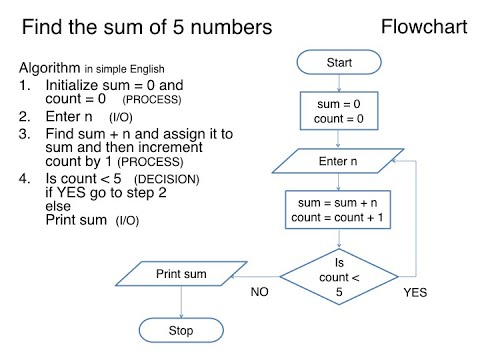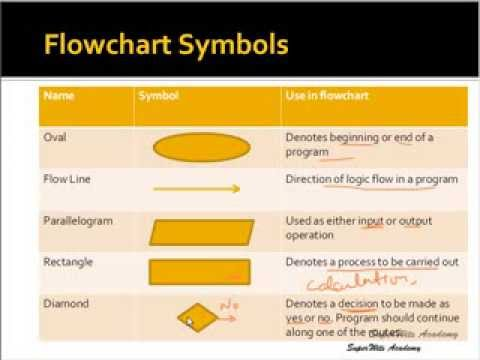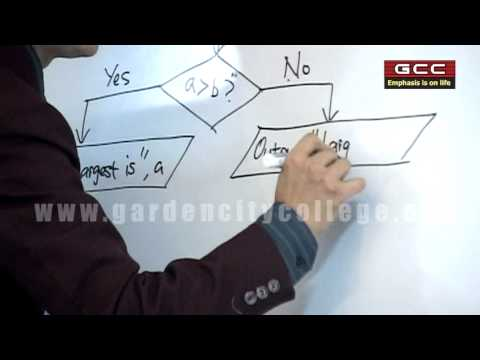## Algorithm, Flowchart and Its Types

Subject: Computer

#### Overview

Algorithm is defined as a set of rules that define how a particular problem can be solved in finite number of steps. This note contains description on how to write algorithm, flowchart and its types.
##### Algorithm, Flowchart and Its Types

We know that computer is user dependent machine. It doesn't work or think on its own. All the activities of computer system are controlled and processed by the instructions and information provided by the user. These instruction are called commands. Collection of such instruction and the data in a systematic form to solve the problem is called the program.
To solve the problem using computer we have to make some systematic plan of action. Such plan of action can be represented in the systematic order using different tools and technique called the program presentation tools and technique. Popular tools and technique used to represent the programs are flowchart and algorithm.

#### Algorithm

Algorithm is defined as a set of rules that define how a particular problem can be solved in finite number of steps. In another word, algorithm is a step by step representation of instructions to solve the problem. An algorithm must be composed of a finite set of steps, each of which may require one or more operations.
The good algorithm should have following features:• Input: specified and required input values.
• Output: outcome values or solution of the problem.
• Definite: What should be done must be clearly defined.
• Effective: In finite amount of time it must be able to perform each step in finite amount.
• Finite: There must be finite number of steps or operations.
• Correct: Desirable and correct output must be able to generate.

#### Flowchart

The step by step solution of a problem in a picture form is known as flowchart. It is one of the most important and oldest techniques to depict an algorithm.
A Flowchart facilitates the computer in following ways:

• Helps to understand program more easily.
• Helps to define input, output and processing sections of program.
• Helps to easily debug the program and act as analytical tool.
• Represents complex algorithm in concise form of documentation.

The basic flowchart symbols are:#### Types of Flow Chart

According to use and application of the flowchart we have two types of flowcharts: system flowchart and program flowchart.

1. System Flowchart: It describes the sequence of processes to perform specific work. It explains the total flow of data inside the system.
2. Program Flowchart: To represent the program, we have to use standard diagram called program flowchart. It shows the flow of instructions to solve the problems. It is used to make logical structure diagram of program.

#### Rules of developing flowchart

1. Analyse the input, process, storage and output of the program.
2. Use standard symbols and arrowhead to the direction of flow of data and instructions.
3. We should use easy word which can be easily understood by other programmer.
4. There should be a list of activities inside each symbol.
5. It should express all sections like input, process, logical and output.
6. It should not be any programming language oriented.

• It is easy method of communication to represent program.
• It serves as a guide for program coding.
• It is easier to understand than program and algorithm.
• It is program independent so applicable for all types of programming languages.
• It represent all the components of program like input, process, logic and output which makes easy to develop the program.

• It cannot replace all types of logic used on the computer program.
• It takes long time to represent all module of computer program.
• It is not suitable and be complex for big problem.
##### Things to remember
• Algorithm is defined as a set of rules that define how a particular problem can be solved in finite number of steps.
• The step by step solution of a problem in a picture form is known as flowchart.
• System flowchart describes the sequence of processes to perform specific work.
• To represent the program, we have to use standard diagram called program flowchart.
• It includes every relationship which established among the people.
• There can be more than one community in a society. Community smaller than society.
• It is a network of social relationships which cannot see or touched.
• common interests and common objectives are not necessary for society.
##### Videos for Algorithm, Flowchart and Its Types##### Algorithm using Flowchart and Pseudo code Level 1 Flowchart##### Computer,Algorithm and Flowchart (Lesson 1)##### Concepts of Algorithm, Flow Chart & C Programming

An algorithm is a sequence of steps required to solve a particular problem. The concept of algorithm was given by ALKHOWARISMI.

1. Some characteristic of an algorithm are listed below:
2. Each and every instruction should be precise and unambiguous.
3. An algorithm should have finite number of steps.
4. An algorithm should produce correct result.
5. An algorithm should not use particular programming language.
Flowchart is a graphical representation of program solving steps so that it makes clear about the program. The concept of flowchart was given by John Vonn Neuman 1945.
Types of flowchart are:
a) System Flowchart b) Program Flowchart
 System flowchart Program flowchart 1. System flowchart gives complete processing mechanism and cannot be converted into program 1. Program flowchart gives the problem solving method and can be converted into program. Example: Organizational structure of a college. Example: Problem to calculate area of triangle.
The common guidelines (properties) of flowchart are:
The flowchart should have only one start and ending points.
Flow lines shouldn't intersect each other.
Flowchart should not contain the programming language.
The flowchart should be neat and clear for the user.

Some of the symbols used in flowchart along with their meanings are listed below:1. It takes long time to prepare to proper flowchart.
2. Translation of flowchart into program is sometimes difficult.

lgorithm

Step 1 : Start

Step 3 : Assign value of pie to P

Step 4 : Find area and circumference

Step 5 : Display area and circumference

Step 6 : StopAlgorithm.

Step 1 : Start

Step 2 : Read A, B

Step 3 : Assign A to X and B to Y

Step 4 : Divide A by B.

Step 5 : Is remainder zero? If yes, go to step 7.

Step 6 : Assign A to B and B to remainder. And go to step 4

Step 7 : Assign B to HCF

Step 8 : Multiply X and Y and divide by HCF. And assign result to LCM.

Step 9 : Display HCF and LCM

Step 10 : Stop